Share

Books Shortlist
Your shortlist is empty

# NCERT solutions for Class 9 Mathematics chapter 12 - Heron's Formula

## Mathematics Class 9

#### NCERT Mathematics Class 9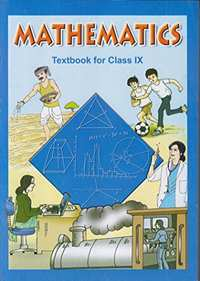## Chapter 12: Heron's Formula

Ex. 12.10Ex. 12.20

#### Chapter 12: Heron's Formula Exercise 12.10 solutions [Pages 202 - 203]

Ex. 12.10 | Q 1 | Page 202

A traffic signal board, indicating ‘SCHOOL AHEAD’, is an equilateral triangle with side ‘a’. Find the area of the signal board, using Heron’s formula. If its perimeter is 180 cm, what will be the area of the signal board?

Ex. 12.10 | Q 2 | Page 202

The triangular side walls of a flyover have been used for advertisements. The sides of the walls are 122m, 22m, and 120m (see the given figure). The advertisements yield an earning of Rs 5000 per m2per year. A company hired one of its walls for 3 months. How much rent did it pay?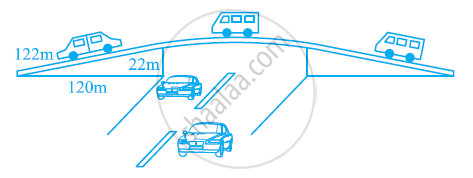Ex. 12.10 | Q 3 | Page 203

There is a slide in the park. One of its side walls has been painted in the same colour with a message “KEEP THE PARK GREEN AND CLEAN” (see the given figure). If the sides of the wall are 15m, 11m, and 6m, find the area painted in colour.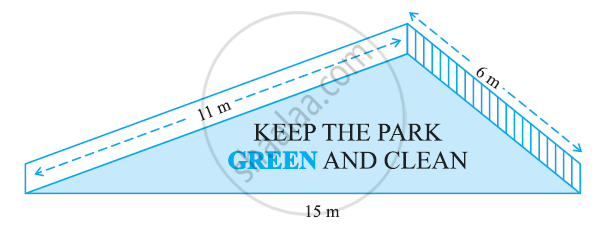Ex. 12.10 | Q 4 | Page 203

Find the area of a triangle two sides of which are 18 cm and 10 cm and the perimeter is 42 cm.

Ex. 12.10 | Q 5 | Page 203

Sides of a triangle are in the ratio of 12: 17: 25 and its perimeter is 540 cm. Find its area.

Ex. 12.10 | Q 6 | Page 203

An isosceles triangle has perimeter 30 cm and each of the equal sides is 12 cm. Find the area of the triangle.

#### Chapter 12: Heron's Formula Exercise 12.20 solutions [Pages 206 - 207]

Ex. 12.20 | Q 1 | Page 206

A park, in the shape of a quadrilateral ABCD, has ∠C = 90°, AB = 9 m, BC = 12 m, CD = 5 m and AD = 8 m. How much area does it occupy?

Ex. 12.20 | Q 2 | Page 206

Find the area of a quadrilateral ABCD in which AB = 3 cm, BC = 4 cm, CD = 4 cm, DA = 5 cm and AC = 5 cm.

Ex. 12.20 | Q 3 | Page 206

Radha made a picture of an aeroplane with coloured papers as shown in the given figure. Find the total area of the paper used.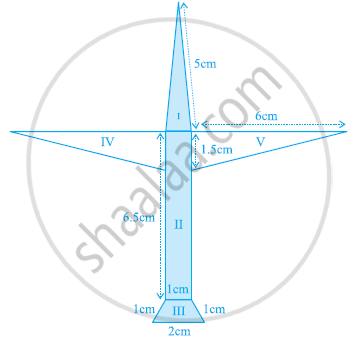Ex. 12.20 | Q 4 | Page 206

A triangle and a parallelogram have the same base and the same area. If the sides of triangle are 26 cm, 28 cm and 30 cm, and the parallelogram stands on the base 28 cm, find the height of the parallelogram.

Ex. 12.20 | Q 5 | Page 207

A rhombus shaped field has green grass for 18 cows to graze. If each side of the rhombus is 30 m and its longer diagonal is 48 m, how much area of grass field will each cow be getting?

Ex. 12.20 | Q 6 | Page 207

An umbrella is made by stitching 10 triangular pieces of cloth of two different colours (see the given figure), each piece measuring 20 cm, 50 cm and 50 cm. How much cloth of each colour is required for the umbrella?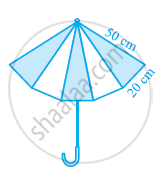Ex. 12.20 | Q 7 | Page 207

A kite in the shape of a square with a diagonal 32 cm and an isosceles triangles of base 8 cm and sides 6 cm each is to be made of three different shades as shown in the given figure. How much paper of each shade has been used in it?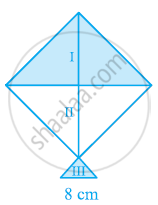Ex. 12.20 | Q 8 | Page 207

A floral design on a floor is made up of 16 tiles which are triangular, the sides of the triangle being 9 cm, 28 cm and 35 cm (see the given figure). Find the cost of polishing the tiles at the rate of 50p per cm2.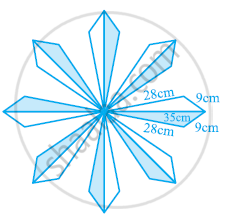Ex. 12.20 | Q 9 | Page 207

A field is in the shape of a trapezium whose parallel sides are 25 m and 10 m. The non-parallel sides are 14 m and 13 m. Find the area of the field.

## Chapter 12: Heron's Formula

Ex. 12.10Ex. 12.20

#### NCERT Mathematics Class 9## NCERT solutions for Class 9 Mathematics chapter 12 - Heron's Formula

NCERT solutions for Class 9 Maths chapter 12 (Heron's Formula) include all questions with solution and detail explanation. This will clear students doubts about any question and improve application skills while preparing for board exams. The detailed, step-by-step solutions will help you understand the concepts better and clear your confusions, if any. Shaalaa.com has the CBSE Mathematics Class 9 solutions in a manner that help students grasp basic concepts better and faster.

Further, we at Shaalaa.com are providing such solutions so that students can prepare for written exams. NCERT textbook solutions can be a core help for self-study and acts as a perfect self-help guidance for students.

Concepts covered in Class 9 Mathematics chapter 12 Heron's Formula are Application of Heron’S Formula in Finding Areas of Quadrilaterals, Area of a Triangle by Heron’S Formula, Introduction to Area of a Triangle.

Using NCERT Class 9 solutions Heron's Formula exercise by students are an easy way to prepare for the exams, as they involve solutions arranged chapter-wise also page wise. The questions involved in NCERT Solutions are important questions that can be asked in the final exam. Maximum students of CBSE Class 9 prefer NCERT Textbook Solutions to score more in exam.

Get the free view of chapter 12 Heron's Formula Class 9 extra questions for Maths and can use Shaalaa.com to keep it handy for your exam preparation

S